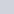Student Forums CMA Part 2 Section C: Decision Analysis C.1. CVP Analysis Question ID: HTB 2.2.014 (Topic: CVP Analysis)

# Question ID: HTB 2.2.014 (Topic: CVP Analysis)

• This topic has 1 reply, 2 voices, and was last updated 1 month ago byLynn Roden.
• Creator
Topic
• #235564Manish Srivastava
Participant

Dear Sir

I got the answer correct but i have a doubt to be cleared. While i took the weighted Average Contribution Margin as 6.75 and divided total FC \$975000 by \$ 6.76 it gave the answer as 144,445 units BEP. i wanted to see the individual units produced by each product line. Therefore i divided FC for Plastic frame \$ 195,000 by \$1.5 (wt. Average Contribution margin ) but the answer was higher than the total BEP units. Please explain

Viewing 1 replies (of 1 total)
• Author
Replies
• #235766Lynn Roden
HOCK international

Hello Manish Srivastava,

If you want to calculate how many of each product needs to be sold in order to break even, you need to apply the existing sales mix of 25% plastic frames and 75% glass frames to the total breakeven volume. Multiply the total breakeven volume by 25% to find the number of plastic frames and multiply the breakeven volume by 75% to find the number of glass frames.

 Plastic: 144,445 x 0.25 = 36,111 Glass: 144,445 x 0.75 = 108,334 144,445

Proof:

 Plastic revenue 36,111 x \$6 = \$216,666 Glass revenue 108,334 x \$7 = 758,338 Contribution margin \$975,004 Less: Fixed costs 975,000 Net income 4

The \$4 difference is due to rounding.

Lynn

Viewing 1 replies (of 1 total)
• You must be logged in to reply to this topic.